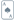Select Page

Number Magic Math Trick Tutorial – Self Working

### A Card Trick

Materials Needed:

– 1 Standard Card Deck with all Tens, Jacks, Queens, and Kings removed. – Ability to do math in your head. – Pad of paper and pencil.How the Trick Appears:

## Math Trick Video Tutorial

Using math you figure out two cards that a person has chosen.How the Trick Works:

Have someone shuffle the cards. Take a group of cards and hold them in your hand, spread out in a fan shape. Ask him/her to choose a card from among them. Tell the person to remember it and then to put it back in the deck.

Give the person a piece of paper and pencil if they are not that good at doing math in their head, or remembering a few numbers at a time.

Ask them to double the value of the card they picked, and then to add 5.

Now have them multiply that number by 5. Tell them to remember this number.

Instruct them to look at another card in the deck, and to add its value to the total that they had computed before. They are to tell you the final total of everything.

In your head, subtract 25 from the total. The two digits you get are the same as the two cards they chose.

Example: they choose a Six (6), then a Three (3).

6 (doubled) = 12 plus 5 = 17. Multiply times 5 = 85.

Three added to total = 88. You subtract 25 = 63.

They picked a Six and a Three!

Notes: Aces are counted as “1”

Practice this trick before performing. It’s easy to get confused on how a card trick works just from reading about it.

Having a deck of cards in your hands as you try and learn the tricks on this page will make it much easy to learn, understand and then master.